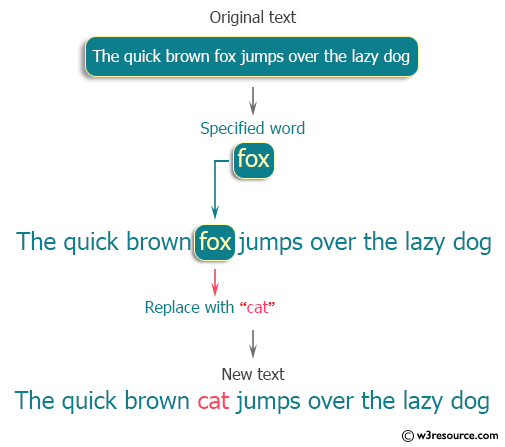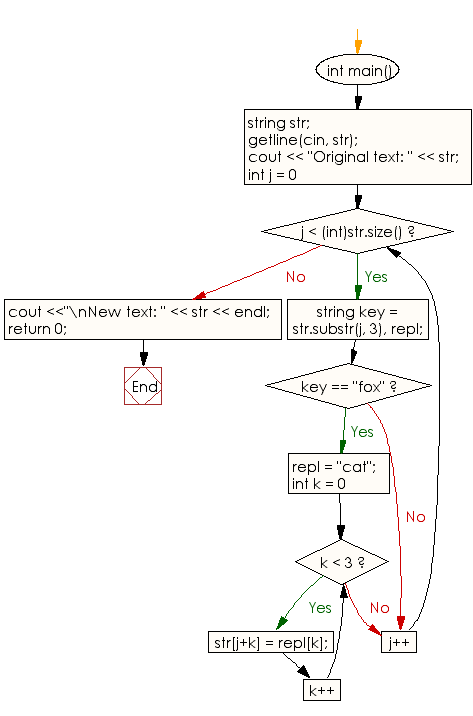﻿ C++ Exercises: Replace all the words "dog" with "cat" - w3resource

# C++ Exercises: Replace all the words "dog" with "cat"

## C++ Basic: Exercise-81 with Solution

Write a C++ program that replaces all the words "dog" with "cat".
Sample Text: The quick brown fox jumps over the lazy dog. You can assume that the number of characters in a text is less than or equal to 1000 .

Pictorial Presentation:Sample Solution:

C++ Code:

``````#include <iostream>
using namespace std;

int main()
{
string str;
getline(cin, str);
cout << "Original text: " << str;
for (int j = 0; j < (int)str.size(); j++) {
string key = str.substr(j, 3), repl;
if (key == "fox") {
repl = "cat";
for (int k = 0; k < 3; k++) {
str[j+k] = repl[k];
}
}
}
cout <<"\nNew text: " << str << endl;

return 0;
}
``````

Sample Output:

```Original text: The quick brown fox jumps over the lazy dog
New text: The quick brown cat jumps over the lazy dog
```

Flowchart:C++ Code Editor:

What is the difficulty level of this exercise?

﻿

## C++ Programming: Tips of the Day

Why is there no std::stou?

The most pat answer would be that the C library has no corresponding "strtou", and the C++11 string functions are all just thinly veiled wrappers around the C library functions: The std::sto* functions mirror strto*, and the std::to_string functions use sprintf.

Ref: https://bit.ly/3wtz2qA

We are closing our Disqus commenting system for some maintenanace issues. You may write to us at reach[at]yahoo[dot]com or visit us at Facebook